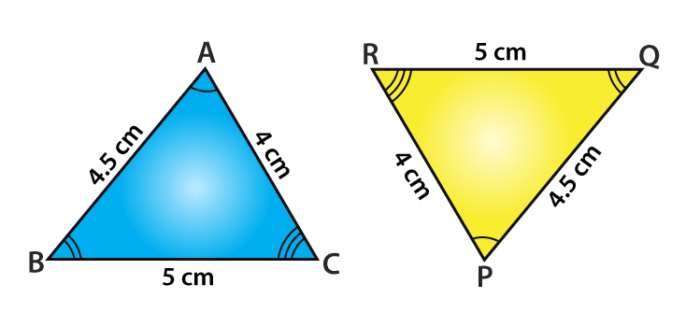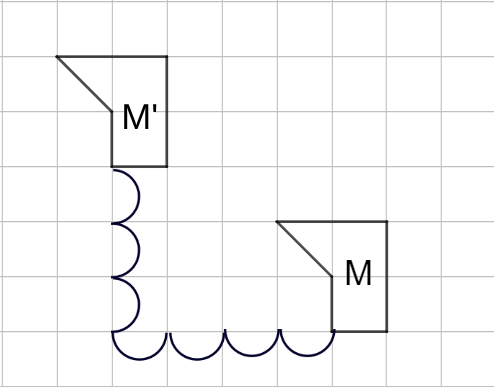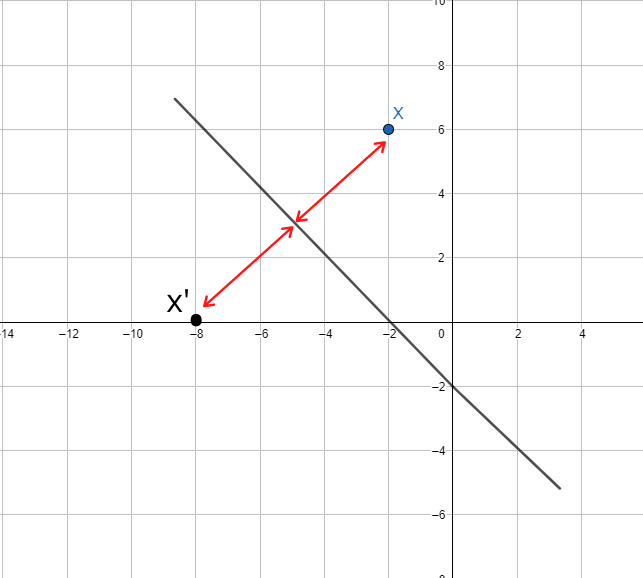## Isometric Transformations

11.1  Transformations

 Definition Transformation involves the transfer of a position on a plane. It also defined as a movement with a certain orientation and match without changing the shape.

 Congruence: Two objects are congruent if both have the same size and shape, regardless of the orientation of the movement. ExampleBoth triangles are congruent as they are of the same size, although the position and orientation are not the same.
11.2  Translation

 Definition The transfer of all points on a plane in the same direction and through the same distance.

Representation of translation in the form of vector:

• Vector of translation is a movement that has direction and magnitude and is determined based on the value and direction of a vector.

Translation can be described using two methods,

(i)

The direction of movement: to the right, left, upwards or downwards

The direction of distance: number of units

(ii)

Written in a vector form $$\dbinom{a}{b}$$

Image and object under a translation:

Example• $$M'$$ is the image of object $$M$$.
• The translation is $$\dbinom{-4}{3}$$.

Determining the coordinate of the image when coordinate of the object is given:

• To locate the image with translation $$\dbinom{a}{b}$$, the coordinate of object $$(x,y)$$ will be mapped to image $$(x+a, y+b)=(x',y')$$.

Two alternative methods are:

• $$\dbinom{a}{b} + \dbinom{x}{y} = \dbinom{a+x}{b +y}$$

• $$\dbinom{a}{b} - \dbinom{x}{y} = \dbinom{a-x}{b -y}$$

Determining the coordinate of the object when the coordinate of the image is given:

• To locate an object with translation $$\dbinom{a}{b}$$, the coordinate of image $$(x',y')$$ will be mapped to object $$(x'-a, y'-b)=(x,y)$$.

The alternative method is:

• $$\dbinom{x}{y} = \dbinom{x'}{y'} - \dbinom{a}{b}$$

Defining vector translation when the position of image and object is given:

Given object $$(x,y)$$ and the image $$(x',y')$$.

$$\text{Vector Translation} = \dbinom{x' -x}{y' -y}$$

11.3  Reflection

 Definition A transformation that occurs when all the points on the plane are reversed in the same plane on a line The line is called the axis of reflection

 Features of Reflections (i) The object and the image are on the opposite sides of the axis of reflection. (ii) The object and its image have the same perpendicular distance from the axis of reflection. (iii) The shape and size of the image is the same as the object, but the orientation is reversed. (iv) The image of a point on the axis of reflection is the point itself. Properties of an Image of a Reflection (i) The image is the same shape and the same size as the object. (ii) The image has different orientations, inverted sides and forms mirror images with one another. Example$$X'$$ is the image of $$X$$ that reflected on the axis of reflection.

11.4   Rotation

 Definition The process of transformation occurs when each point is rotated at a fixed point through a certain angle and in a certain direction.

 Properties of Rotation (i) The resulting image has the same shape, size and orientation as the object. (ii) The centre of rotation is a stationary point. (iii) The distance of all the points of the image to the centre of the rotation is equal to the distance of the object to the centre of rotation.

11.5  Translation, Reflection and Rotation as an Isometry

 Relationship between translation, reflection and rotation as isometry: If an object is mapped to a congruent image, then it is an isometry. Isometry is a transformation that maintains the distance between any two points on the original object. The isometric transformation will retain the original shape and size of the object. Relationship between the isometry and congruence: Under an isometry, objects and images are of equal shape and size. Therefore, objects and images are congruent. Isometry is a transformation where the image is congruent with the object.
11.6  Rotational Symmetry

• The shape or image is rotated in less than $$360^{\circ}$$ at a fixed point.
• Its shape will remain the same.

Order of rotational symmetry of an object:

• The order of rotational symmetry is the number of times a shape moves to return to its original shape in a complete rotation.
• The order of rotational symmetry is equal to the number of axes of symmetry of an object.
###### Topic : Transformation
Form 2 Mathematics

Report this note